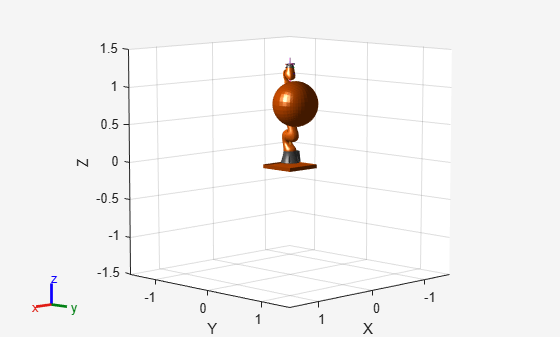Main Content

# interpolate

Interpolate states along path from RRT

## Syntax

``interpPath = interpolate(rrt,path)``
``interpPath = interpolate(rrt,path,numInterp)``

## Description

example

````interpPath = interpolate(rrt,path)` interpolates states between each adjacent configuration in the path based on the ValidationDistance property of the manipulator rapidly exploring random tree (RRT) planner `rrt`.```
````interpPath = interpolate(rrt,path,numInterp)` specifies the number of interpolations between adjacent configurations.```

## Examples

collapse all

Use the `manipulatorRRT` object to plan a path for a rigid body tree robot model in an environment with obstacles. Visualize the planned path with interpolated states.

Load a robot model into the workspace. Use the KUKA LBR iiwa© manipulator arm.

`robot = loadrobot("kukaIiwa14","DataFormat","row");`

Generate the environment for the robot. Create collision objects and specify their poses relative to the robot base. Visualize the environment.

```env = {collisionBox(0.5, 0.5, 0.05) collisionSphere(0.3)}; env{1}.Pose(3, end) = -0.05; env{2}.Pose(1:3, end) = [0.1 0.2 0.8]; show(robot); hold on show(env{1}) show(env{2})```Create the RRT planner for the robot model.

`rrt = manipulatorRRT(robot,env);`

Specify a start and a goal configuration.

```startConfig = [0.08 -0.65 0.05 0.02 0.04 0.49 0.04]; goalConfig = [2.97 -1.05 0.05 0.02 0.04 0.49 0.04];```

Plan the path. Due to the randomness of the RRT algorithm, set the `rng` seed for repeatability.

```rng(0) path = plan(rrt,startConfig,goalConfig);```

Visualize the path. To add more intermediate states, interpolate the path. By default, the `interpolate` object function uses the value of `ValidationDistance` property to determine the number of intermediate states. The `for` loop shows every 20th element of the interpolated path.

```interpPath = interpolate(rrt,path); clf for i = 1:20:size(interpPath,1) show(robot,interpPath(i,:)); hold on end show(env{1}) show(env{2}) hold off```## Input Arguments

collapse all

Manipulator RRT planner, specified as a `manipulatorRRT` object. This planner is for a specific rigid body tree robot model stored as a `rigidBodyTree` object.

Planned path in joint space, specified as an r-by-n matrix of joint configurations, where r is the number of configurations in the path, and n is the number of nonfixed joints in the `rigidBodyTree` robot model.

Data Types: `double`

Number of interpolations between each configuration, specified as a positive integer.

Data Types: `double`

## Output Arguments

collapse all

Planned path in joint space, specified as an r-by-n matrix of joint configurations, where r is the number of configurations in the path and n is the number of nonfixed joints in the `rigidBodyTree` robot model.

Data Types: `double`

## Extended Capabilities

### C/C++ Code GenerationGenerate C and C++ code using MATLAB® Coder™.

Introduced in R2020b